# Selberg Conjectures

Selberg has made two conjectures concerning the Dirichlet series in the Selberg class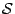:

Conjecture A. For each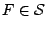there exists an integer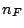such that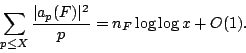Conjecture A follows from

Conjecture B. Ifis primitive, then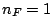, and if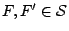are distinct and primitive, then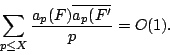The above sums are over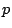prime.

Conjecture B can be interpreted as saying that the primitive functions form an orthonormal system. This conjecture is very deep. It implies, among other things, Artin's conjecture on the holomorphy of non-abelian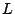-functions [ MR 98h:11106], and that the factorization of elements into primitives is unique [ MR 95f:11064].

If you extend the Selberg Class to include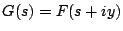forand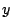real, then Conjecture B with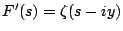is equivalent to a prime number theorem for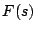.

Back to the main index for The Riemann Hypothesis.